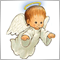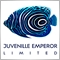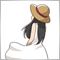# Multi-timeframe Fractals on same chart?261

Hi,

Is there any way to plot the Fractals indicator on a different time frame from the current chart?

I have tried replacing the High[i] section with the iHigh function with iHigh(NULL,PERIOD_H4,0)[i]. However it doesn't seem to work and says an "array is missing [".

I am basically looking for it to plot multiple timeframe fractals on the one chart. Any ideas?

Thanks :)3010

torexvir:

Hi,

Is there any way to plot the Fractals indicator on a different time frame from the current chart?

I have tried replacing the High[i] section with the iHigh function with iHigh(NULL,PERIOD_H4,0)[i]. However it doesn't seem to work and says an "array is missing [".

I am basically looking for it to plot multiple timeframe fractals on the one chart. Any ideas?

Thanks :)

iHigh(NULL,PERIOD_H4,i)

Pay attention to right "i". It must belong to the right timeframe (to PERIOD_H4 in this case).261

Petr Nosek:

iHigh(NULL,PERIOD_H4,i)

Pay attention to right "i". It must belong to the right timeframe (to PERIOD_H4 in this case).

Of course!!!

Totally didn't spot that somehow ha. I'll give it a try.

Thank you 😁261

```#property indicator_chart_window
#property indicator_buffers 2
#property indicator_color1 Red
#property indicator_color2 Blue
//---- input parameters

//---- buffers
double ExtUpFractalsBuffer[];
double ExtDownFractalsBuffer[];

//+------------------------------------------------------------------+
//| Custom indicator initialization function                         |
//+------------------------------------------------------------------+
int init()
{

//---- indicator buffers mapping
SetIndexBuffer(0,ExtUpFractalsBuffer);
SetIndexBuffer(1,ExtDownFractalsBuffer);
//---- drawing settings
SetIndexStyle(0,DRAW_ARROW);
SetIndexArrow(0,119);
SetIndexStyle(1,DRAW_ARROW);
SetIndexArrow(1,119);
//----
SetIndexEmptyValue(0,0.0);
SetIndexEmptyValue(1,0.0);
//---- name for DataWindow
SetIndexLabel(0,"Fractal Up");
SetIndexLabel(1,"Fractal Down");

//---- initialization done
return(0);
}
//+------------------------------------------------------------------+
//| Custor indicator deinitialization function                       |
//+------------------------------------------------------------------+
int deinit()
{

//----
return(0);
}
//+------------------------------------------------------------------+
//| Custom indicator iteration function                              |
//+------------------------------------------------------------------+
int start()
{

int    i,nCountedBars;
bool   bFound;
double dCurrent;
nCountedBars=IndicatorCounted();
//---- last counted bar will be recounted
if(nCountedBars<=2)
i=iBars(NULL,PERIOD_H4)-nCountedBars-3;
if(nCountedBars>1)       //changed this from 2
{
nCountedBars--;
i=iBars(NULL,PERIOD_H4)-nCountedBars-1;
}
//----Up and Down Fractals
while(i>=2)
{
//----Fractals up
bFound=false;
dCurrent=iHigh(NULL,PERIOD_H4,i);
if(dCurrent>iHigh(NULL,PERIOD_H4,i+1) && dCurrent>iHigh(NULL,PERIOD_H4,i-1));   //took these out dCurrent>High[i+2]&& dCurrent>High[i-2]
{
bFound=true;
ExtUpFractalsBuffer[i]=dCurrent;

}

//----Fractals down
bFound=false;
dCurrent=iLow(NULL,PERIOD_H4, i);
if(dCurrent<iLow(NULL,PERIOD_H4,i+1)&& dCurrent<iLow(NULL,PERIOD_H4, i-1)); //took this out dCurrent<Low[i+2]&& dCurrent<Low[i-2
{
bFound=true;
ExtDownFractalsBuffer[i]=dCurrent;
}

i--;
}
//----
return(0);
}
//+------------------------------------------------------------------+```
Petr Nosek

:

iHigh(NULL,PERIOD_H4,i)

Pay attention to right "i". It must belong to the right timeframe (to PERIOD_H4 in this case).

So i made the changes and the bugs disappeared. Made some other changes to account for the fact i am retrieving counted bars as iBars now and not simply Bars. However all i am getting now is the highs and lows of every candle on the 4hr timeframe plotted. Im basically just looking for the 4hr fractals plotted on any other timeframe?

Thanks

This is the code^^^^Moderator
10190261

Eleni Anna Branou: